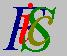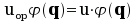Mauro Murzi's pages on Philosophy of Science - Quantum mechanics
State description in quantum mechanicsBohr energy levels Second Schrödinger equation# [3. Schrödinger equations.]

## State description in quantum mechanics.

The state S of a quantum system is completely described by a complex function Ψ (q) depending from position q.

Let u be a classical physical quantity and let uop be the corresponding quantum operator. The first Schrödinger equation is. The eigenvalues ui are the admissible values of u.

The complex function Ψ (q) can be represented as a sum of the eigenfunctions:Complex numbers σi are called the expansion coefficients; the square of their module determines the probability PSui ) that u has the value ui in the state S :   PSui ) = | σi |² . The square of the module of Ψ (q) gives the probability that, in the state S, the system is to be found in position q :   PSq ) = | Ψ (q) |² .

The probability of every physical quantity, in the state S, is determined by the complex function Ψ (q) together with the first Schrödinger equation, by means of the following two steps:

1. The eigenvalues of the first Schrödinger equation give the admissible values for that physical quantity.
2. The representation of Ψ (q) as a sum of the eigenfunctions gives, by means of the expansion coefficients, the probability of every value.Bohr energy levels Second Schrödinger equation